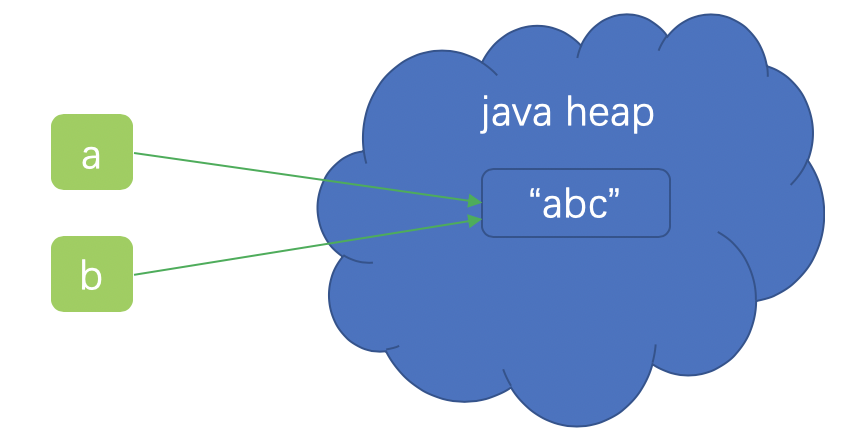# 简介

String是java中非常常用的一个对象类型。可以说java中使用最多的就是String了。那么String到底有哪些秘密呢？接下来本文将会一一讲解。

# String是不可变的

String是不可变的，官方的说法叫做immutable或者constant。

JDK9之前，String的底层其实是一个Char的数组。

``````private final char value[];
``````

`````` private final byte[] value;
``````

``````String a="abc";
String b="abc";
````````````String c= new String("abc");
``````

``````    public String(String original) {
this.value = original.value;
this.hash = original.hash;
}
``````

String的不可变性还在于，String的所有操作都会产生新的字符串字面量。原来的字符串是永远不会变化的。

# 传值还是传引用

``````public static void main(String[] args) {
String x = new String("ab");
change(x);
System.out.println(x);
}

public static void change(String x) {
x = "cd";
}
``````

# substring() 导致的内存泄露

``````String(int offset, int count, char value[]) {
this.value = value;
this.offset = offset;
this.count = count;
}

public String substring(int beginIndex, int endIndex) {
//check boundary
return  new String(offset + beginIndex, endIndex - beginIndex, value);
}
``````

``````String string = "abcdef";
String subString = string.substring(1, 3);
string = null;
``````

``````public String(char value[], int offset, int count) {
//check boundary
this.value = Arrays.copyOfRange(value, offset, offset + count);
}

public String substring(int beginIndex, int endIndex) {
//check boundary
int subLen = endIndex - beginIndex;
return new String(value, beginIndex, subLen);
}
``````

Arrays.copyOfRange将会拷贝一份新的数组，而不是使用之前的数组。从而不会发生上面的内存泄露的问题。